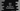# Java example program to find the area of a Parallelogram## Java example program to find the area of a Parallelogram:

In this post, we will learn how to find the area of a parallelogram. It is a quadrilateral with two parallel sides. Opposite sides are of equal length and opposite angles are of equal size.

The area of a parallelogram can be calculated by using the below formula:

``area = height x base``

So, if we can get the height and base of the parallelogram, we can calculate the area.

The height of a parallelogram is the distance between its opposite sides.base is any one of its side, but a base and height should perpendicular to each other.

Our program will take the height and base of the parallelogram as inputs from the user and it will print the area.

## Java program:

Let’s write down the program now:

``````import java.util.Scanner;

class Main {
public static void main(String[] args) {
Scanner scanner = new Scanner(System.in);
float height, base, area;

System.out.println("Enter the height size: ");
height = scanner.nextFloat();

System.out.println("Enter the base size: ");
base = scanner.nextFloat();

area = height * base;

System.out.println("Area: " + area);
}
}``````

Here,

• scanner is the Scanner variable. This is used to read user input values. We need to import java.util.Scanner to use it.
• height, base and area are three floating point variables to store the values of height, base and area.
• It asks the user to enter the height and stores that value in the variable height. Similarly, it reads and stores the base value in base. Then, it finally calculates the area value by multiplying these values.
• The last line is printing the area.

If you run this program, it will print one output as like below:

``````Enter the height size:
5
Enter the base size:
5
Area: 25.0``````

### Find the area by using a function:

We can also create one different function to calculate the area. The advantage of this approach is that you can call this method from many places in your application. If you have 100s of files and you need to calculate the area in multiple places, you can define it in a method in any utility class and call that method from anywhere you want.

Let’s change the above program to use a different function:

``````import java.util.Scanner;

class Main {
public static float findArea(float height, float base){
return height * base;
}
public static void main(String[] args) {
Scanner scanner = new Scanner(System.in);
float height, base, area;

System.out.println("Enter the height size: ");
height = scanner.nextFloat();

System.out.println("Enter the base size: ");
base = scanner.nextFloat();

area = findArea(height, base);

System.out.println("Area: " + area);
}
}``````

Here, findArea is a different function that takes height and base as the parameters and returns the area. The return value is float and this method is public static. So, we can call it from any other classes as well without creating a new object of Main.